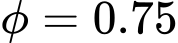﻿ Verification of Rectangular RC Cross-Section | AS 3600 - 2018 | GEO5 | Online nápověda

Odkaz jsme Vám odeslali na email.

Nepodařilo se nám odeslat odkaz na váš email. Zkontrolujte, prosím, Váš email.

# Online nápověda

Stromeček
Nastavení
Produkt:
Program:
Jazyk:

## Verification of Rectangular RC Cross-Section

The cross-section is rectangular, unilaterally reinforced and loaded by the bending moment and normal compression force. The program verifies a reinforced concrete section using the method of limit deformation (Art. 8.1). The maximum allowable strain of concrete in compression is 0.003. Compression reinforcement is not taken into account.

The computed degree of reinforcement is checked using the following expressions: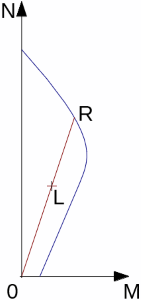Interaction diagram N-M

Usage ratio of concrete cross-section subject to the combination of bending moment and normal force is determined as |0L| / |0R|. Where L is load and R is strength with prescribed excentricity.

## Bending without normal force

The cross-section is rectangular, reinforced on one side and loaded by the bending moment M.

The permissible moment for a given area of reinforcements As reads: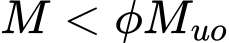for L class reinforcement (Art. 2.2.2):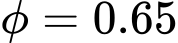for N class reinforcement:kde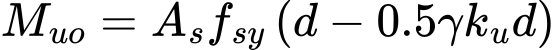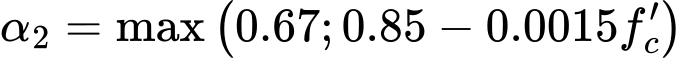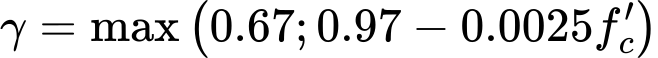The program further checks whether the neutral axis parameter ku is less than the limit value (Art 8.1.5) :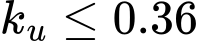## Shear

First, the program computes the ultimate shear strength of concrete Vuc (Art. 8.2.4.1).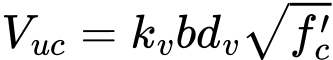where (Art. 8.2.1.9, Art. 8.2.4.3):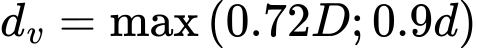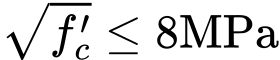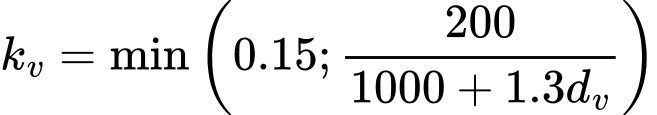If the ultimate shear strength of concrete is exceeded, the ultimate shear strength Vu,max is checked (Art. 8.2.3.3).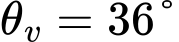Next, the necessary reinforcement area is given by (Art. 8.2.5.2):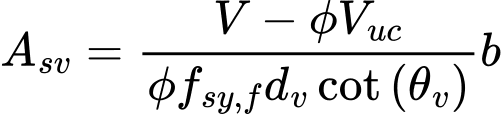where: